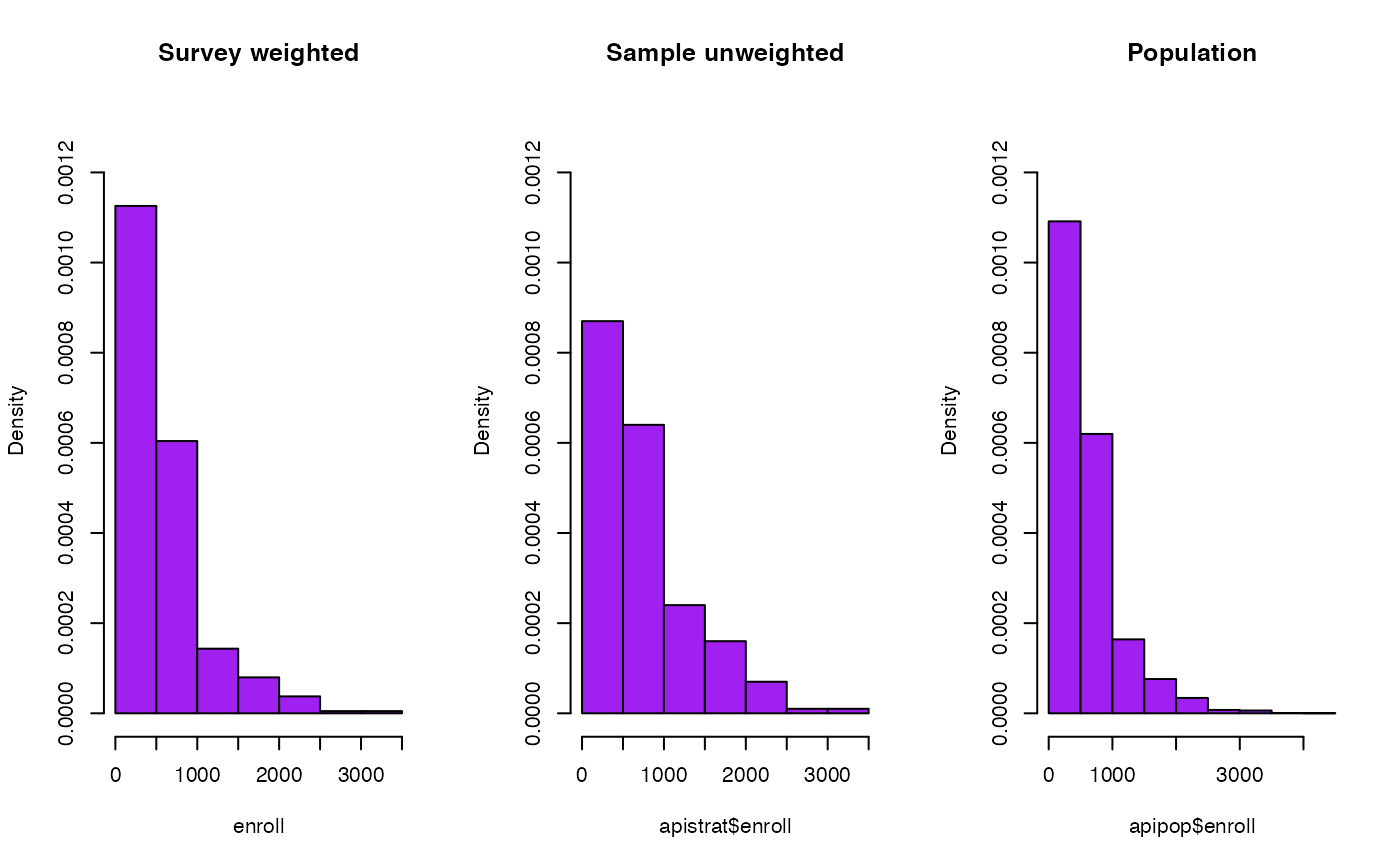Histograms and boxplots weighted by the sampling weights.

svyhist(formula, design, breaks = "Sturges",
include.lowest = TRUE, right = TRUE, xlab = NULL,
main = NULL, probability = TRUE, freq = !probability, ...)
svyboxplot(formula, design, all.outliers=FALSE,...)

## Arguments

formula

One-sided formula for svyhist, two-sided for svyboxplot

design

A survey design object

xlab

x-axis label

main

Main title

probability,freq

Y-axis is probability density or frequency

all.outliers

Show all outliers in the boxplot, not just extremes

breaks, include.lowest, right

As for hist

...

Other arguments to hist or bxp

## Details

The histogram breakpoints are computed as if the sample were a simple random sample of the same size.

The grouping variable in svyboxplot, if present, must be a factor.

The boxplot whiskers go to the maximum and minimum observations or to 1.5 interquartile ranges beyond the end of the box, whichever is closer. The maximum and minimum are plotted as outliers if they are beyond the ends of the whiskers, but other outlying points are not plotted unless all.outliers=TRUE. svyboxplot requires a two-sided formula; use variable~1 for a single boxplot.

## Value

As for hist, except that when probability=FALSE, the return value includes a component

count_scale giving a scale factor between density and counts, assuming equal bin widths.

svyplot

## Examples

data(api)
dstrat <- svydesign(id = ~1, strata = ~stype, weights = ~pw, data = apistrat,
fpc = ~fpc)
opar<-par(mfrow=c(1,3))
svyhist(~enroll, dstrat, main="Survey weighted",col="purple",ylim=c(0,1.3e-3))
hist(apistrat$enroll, main="Sample unweighted",col="purple",prob=TRUE,ylim=c(0,1.3e-3)) hist(apipop$enroll,  main="Population",col="purple",prob=TRUE,ylim=c(0,1.3e-3))par(mfrow=c(1,1))
svyboxplot(enroll~stype,dstrat,all.outliers=TRUE)svyboxplot(enroll~1,dstrat)par(opar)